# Squirrel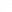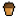Squirrel is a programming language similar to Lua, but uses a C like syntax. In Source, Squirrel is used as one of the scripting languages in the VScript scripting system. The official Squirrel documentation can be found here.

Squirrel heavily uses an associative array data structure called a table. Both the keys and values of a table can contain almost any kind of variable type. The scripting environment consists of nested tables, and when a script is executed its variables and functions are added as table slots.

```printl("Hello World"); // statements can end with both a semicolon...
printl("Hello World")  // ...or a newline character
```

Squirrel's syntax is similar to C/C++/Java etc... but the language has a more dynamic nature like Python/Lua etc. Statements can be separated with both a new line or `;` (or with the keywords `case` or `default` if inside a switch/case statement), both symbols are not required if the statement is followed by `}`.

## File format

### Variables

Squirrel has mainly two kinds of variables: table slots and `local` variables. This doesn't have to do with types; Like in Python, any variable can be of any data type at any time and there are no type declarations. Since the execution scope is always a table, any variables declared outside functions will always be table slots.

• Table slots have to be declared using the `<-` operator. These are to be used for values that should persist when a function ends. Once a slot exists, it can be reassigned with both the `=` or the `<-` operator. The former will throw an exception if the slot does not exist and should be preferred if a reassignment is expected (and not a slot creation).
• Local variables can be declared using the `local` keyword. Each of these is only accessible in the scope it was defined in, namely its surrounding curly brackets. They are to be used temporarily or as helpers that can be discarded later on. A typical example is a `for` loop with an index variable: `for (local i = 0; i < 20; i++) {...}`.
• Global variables are simply table slots in the root table ( `getroottable()` ). They can be declared using the `::` scoping operator and accessed with or without it.
• Constants are declared with the `const` keyword and are intended for values that will never change at runtime, such as Pi or a string representing a file path. Since constants are a compile-time feature, their value is computed compile-time and thus can only be an integer, float or string. Attempting to assign a new value to a constant yields a compile-time error `"can't assign expression"`; It can be imagined that Squirrel replaces each occurrence of the constant's identifier in the code with its current value, hence the error message. Current, because the same constant can be defined multiple times in code but with different values (with the `const` keyword). During runtime, `getconsttable()` returns a table with all constants that have been found during compiling; If more code is compiled, that very table is used to resolve constants. The constants table and its values behave normally and don't have any protection that constants usually have.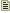Note:`local` and `const` variables are declared with the `=` operator. Using `<-` for those yields a compile time error.
 ```a <- 5 // creates a table slot in this table b <- "A text string" // creates a table slot in this table ::x <- 10 // creates a table slot in the root table local i = 0 // initializes a temporary variable const E = 2.71828 // creates a new constant b = "New string" // modifies the existing table slot x = 11 // modifies the existing table slot ```

The `in` operator can be used to test whether a table slot exists. One could also do: `try {a} catch (e) { /*...*/ }`. The following code is assumed to be executed after the above, i.e. in the same environment.

 ```"a" in this // true "x" in this // false, unless we're in the root table "i" in this // false, local variables aren't table slots "E" in this // false, unless we're in the constants table delete a // deletes a table slot "a" in this // false, no longer exists ```

### Data Types

Squirrel is a dynamically typed language. Any variable can be assigned a value of any type.Note: The VScript API functions are bindings to the Source C++ code, so while it may seem possible to pass values of any type to them, they only accept specific types.

The built-in function `typeof` returns the type of an input value as a string in lower case, e.g. `typeof(5) == "integer"`.

Type Description
`Integer` The integers `-2,147,483,648` to `2,147,483,647`, namely -231 to 231-1.
`Float` `-3.4028235e38` to `3.4028235e38`, namely -3.4028235 × 1038 to 3.4028235 × 1038.

In code, a number becomes a float by adding a decimal point. The notations `1.0` and `1.` are legal whereas `.0` or `0f` cause a compile error.
There are no constants for +infinity, -infinity and NaN, but `1.0/0.0`, `-1.0/0.0` and `0.0/0.0` evaluate to to them, respectively.
When converted to a string using `<float>.tostring()` or `format("%f", <float>)`, they become to:`1.#INF`, `-1.#INF` and `-1.#IND`;`inf`, `-inf` and `nan`.

`String` An immutable string. They behave like C strings, and support escape sequences (`\t,\a,\b,\n,\r,\v,\f,\\,\",\',\0,\xhhhh`). These sequences will not work when used in a verbatim string literal.
`Null` Null data type with the only value `null`.
`Bool` Boolean data type that can be `true` or `false`.
`Table` Associative array.
`Array` Mutable C style array.
`Function` Second order functions are supported, so functions can be assigned as values to variables.
`Class` Object oriented class. Implementation similar to tables.
`Class Instance` Object instances of classes. Script handles generated by the game also identify as instances.
`Generator` A function whose execution can be suspended and resumed.
`Userdata` Opaque data used by the game.
`Thread` A coroutine instance, works similarly to a generator but has it's own execution stack.
`Weak reference` A weak reference will be set to `null` when the object that the reference is pointing at gets destroyed.

#### Bool

Squirrel considers `null`, `0` (type Integer) and `0.0` (type Float) as `false`, any other value is considered `true`. This allows you for example to null-check variables using `if (var)`.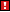Warning:Don't null-check Integers or Floats like this if they may be 0 or 0.0, obviously. This concerns the built-in string function `find(<substring>)` which returns either the index where it found the given substring or null. Do `if (var == null)` instead.
 ```if (false) { /* ... */ } // not executed if (null) { /* ... */ } // not executed if (0) { /* ... */ } // not executed if (0.0) { /* ... */ } // not executed ```

#### Tables

Tables are associative arrays, meaning that they contain a collection of key-value pairs, called slots. A table can be indexed into using the key, returning the associated value. The keys and values can be of any data type (except `null` for the key), including other tables, allowing the creation of more complex data structures.

Tables are defined using curly brackets. Inside a table definition slots can be defined using the `key1 = value1, key2 = value2, ...` pattern. Outside of the definition, new slots can be added to existing tables using the `table.key <- value` pattern.

Using the `.` operator to index a key only works when the key is a string or numerical literal. Array style indexing `table[keyVariable]` can be used to index any kind of key.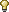Tip:Some of the built-in functions for tables are `len(), clear()`.
 ```// Table defintion. myTable <- { a = 3, // key: "a", value: 3 b = 7, // key: "b", value: 7 x = "example text", // key: "x", value: "example text"  = "six" // key: 6 , value: "six" nestedTable = { z = "another string" } } // alternatively: myTable <- {} myTable["a"] <- 3 // string keys can be indexed... myTable.b <- 7 // ...in two ways myTable.x <- "example text" myTable <- "six" // numbers must be indexed like this myTable.nestedTable <- { z = "another string" } ``` ```foreach (key, value in myTable) { printl(key + ": " + value) } // Prints (order might vary!): // a: 3 // x: example text // b: 4 // 6: six // nestedTable: (table : 0x000000000069D130) printl("a" in myTable) // prints "true" printl( 3 in myTable) // prints "false" printl(myTable.len()) // prints 5 ``` ```// Adding non-string keys a <- "0" myTable[a] <- 11 // key: "0", value: 11 printl(myTable.a) // prints "3" printl(myTable[a]) // prints "11" printl(myTable["0"]) // prints "11" printl(myTable) // exception, key 0 doesn't exist printl(myTable.nestedTable.z) // prints "another string" ```

#### Arrays

Arrays are sequences of objects that are numerically indexed starting from 0. Syntactically they function similarly to C arrays, but unlike C arrays, they are mutable and values can be inserted and removed using a set of built in functions.

Arrays are defined using square brackets.Warning:You can't use the `in` operator to test whether some value is in the array because for Squirrel, arrays are tables where the keys are the indices. The `in` operator would look through the indices that the array has and not its values.Tip:Some of the built-in functions for arrays are `len(), clear(), append(<value>), insert(<index>, <value>), remove(<index>), reverse(), sort([func(a,b)])`.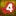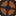In Squirrel 3.x, more functions are available, including `find(<value>), map(<func(a)>) slice(<start>,[end])`.
 ```// Array definition. myArray <- [ 7, // key: 0, value: 7 "more text", // key: 1, value: "more text" null // key: 2, value: null ] // alternatively: myArray <- [] myArray.append(7) myArray.append("more text") myArray.append(null) // alternatively: myArray <- array(3) myArray = 7 myArray = "more text" myArray = null ``` ```foreach (index, value in myArray) { printl(index + ": " + value) } // alternatively: for (local i = 0; i < myArray.len(); i++) { printl(i + ": " + myArray[i]) } // Prints: // 0: 7 // 1: more text // 2: null printl( 2 in myArray) // prints "true" printl("more text" in myArray) // prints "false" printl(myArray.len()) // prints 3 ``` ```printl(myArray) // prints "7" myArray.remove(0) // removes the element at index 0 // and rearranges all other indices printl(myArray) // prints "more text" ```

#### Functions

Functions in Squirrel work similarly to their C counterparts. Second order functions are supported, so functions can be directly manipulated like other values, including it being possible to store them in variables and table slots. Default parameter values and variable numbers of parameters are also supported.

For more details, see the Squirrel API about functions.

It is common practice to begin with a capital letter when naming functions.

 ```// declares and (re)defines "Greet" function Greet() { printl("Hello!") } Greet() // prints "Hello!" ``` ```// declares and (re)defines "Greet" Greet <- function() { printl("Hello!") } Greet() // prints "Hello!" ``` ```// (re)defines "Greet"; exception if "Greet" not declared! Greet = function() { printl("Hello!") } Greet() // prints "Hello!" ``` ```// Functions that consist of one statement // don't need curly brackets "{" and "}" Greet = function() printl("Hello!") Greet() // prints "Hello!" ```

When a function is called, it can be made so that it can or must receive input values that determine the function's side effects and/or its return value.

• Multiple parameters must be comma delimited: `function Test(a, b, c, d = 4, e = 5) {...}`
• All parameters with no default value must be ordered first.
• To avoid exceptions, it is often a good idea to check if a parameter is not `null`.
• As parameters are not restricted to a type, the `typeof` function can be used to handle different types differently.
 ```// normal parameter function Greet(name) { printl("Hello, " + name + "!") } Greet() // exception Greet("Gabe") // prints "Hello, Gabe!" ``` ```// parameter with default value function Greet(name = "Gordon") { printl("Hello, " + name + "!") } Greet() // prints "Hello, Gordon!" Greet("Gabe") // prints "Hello, Gabe!" ```

A function can have a variable number of arguments by specifying `"..."` as last parameter. In the function body, the variable `vargv` will be available and behaves like a normal array.Note:In Squirrel 2.x, `vargv` is a pseudo-array that doesn't behave like a normal array. Every occurrence of it must be indexed with a number such as `vargv`. The length of it is stored in another variable named `vargc` which however does not exist in Squirrel 3.x, making code incompatible.
 ```// Squirrel 3.x varArgFunction <- function(delimiter, ...) { foreach (i, arg in vargv) print(i + delimiter + arg + "\n") } varArgFunction(" : ", 4, null, "string") // prints: // 0 : 4 // 1 : null // 2 : string ``` ```// Squirrel 2.x varArgFunction <- function(delimiter, ...) { for (local i = 0, i < vargc; i++) print(i + delimiter + vargv[i] + "\n") } varArgFunction(" : ", 4, null, "string") // prints: // 0 : 4 // 1 : null // 2 : string ```

A function is ended with the optional `return` statement, where it is also possible to return a value. You can imagine a return value as being 'inserted' into where the function was called. If no return value is specified within the function, it returns the value as `null`.

 ```function IsEven(n) { if (n % 2 == 0) return true else return false } // alternatively: IsEven <- function(n) return n % 2 == 0 if (IsEven(42)) // (42 % 2 == 0) == true { printl("42 is even!") } ```Since Squirrel 3.x, there are lambda expressions which are used as a more convenient way to define a function that just returns a single expression. These are especially useful when a function is needed as a parameter for a different function such as `array.sort` or `array.map`.

 ```local func = @(a,b) a - b // == function(a,b) { return a - b } printl( func(17,9) ) // prints 8 local arr = [1,5,4,6,2,3] arr.sort(func) // arr == [1,2,3,4,5,6] arr = arr.map( @(x) x * x ) // == function(x) { return x * x } // arr == [1,4,9,16,25,36] ```
##### Generators

A generator is a function where the key word `yield` appears; It suspends the execution of that generator function and can return some expression to the function that had resumed it. Some generator `g` can be resumed with the expression `resume g`; The value of this `resume` expression is what was `yield`ed when the function has been suspended the last time. The `return` value of a generator does not matter.

Generators can be used in `foreach` statements, such that no `resume` statement must be typed.

```function PowersOfTwo()
{
for (local n = 1; true; n *= 2)
yield n
}

local x = 100
// Set x to the smallest power of 2
// that is larger than or equal to x:
foreach (i,pow in PowersOfTwo())
{
if (pow >= x)
{
x = pow
break
}
}
```

#### Classes

The following is just an example of some basic class syntax. For details, see Squirrel API about classes.Tip:Some of the metamethods you might want to override include `_tostring(), _typeof(), _cmp(other), _add(other), _sub(other), _mul(other), _div(other), _modulo(other), _nexti(previdx)`.
```class Weapon
{
static prefix = "weapon_" // static variables are read-only!

// For instance-individual variables it's best to declare
// them with anything and overwrite them on construction.
name    = null
primMax = null
secMax  = null

// Containers and instances are not copied per instance, just
// the reference. count is the same for all of instances.
count = 

constructor(name0, prim, sec)
{
name    = prefix + name0
primMax = prim
secMax  = sec
count++
}

function GetEntities()
{
local list = []
local wep = null
while ( wep = Entities.FindByClassname(wep, name) )
{
list.append(wep)
}
return list
}

// ... more useful functions ...
}

awp  <- Weapon("awp", 10, 30)
ak47 <- Weapon("ak47", 30, 90)
// can access class variables and functions
// with awp.primMax, awp.GetEntities(), etc.
```

## Files

With the Source VScript system, scripts are stored in the `game\scripts\vscripts\` directory. Squirrel scripts use the `.nut` file extension, and the `.nuc` extension for ICE encrypted files.

Common to all Squirrel using games, scripts can be associated to a game entity as an entity script that is executed then the entity spawns, and has access to the script handle of its entity through the `self` variable. Specific games can also have other methods of executing scripts.

Script files can also be executed from the Developer Console using the `script_execute` command. Lines of code can even be executed in-line with the `script` command.